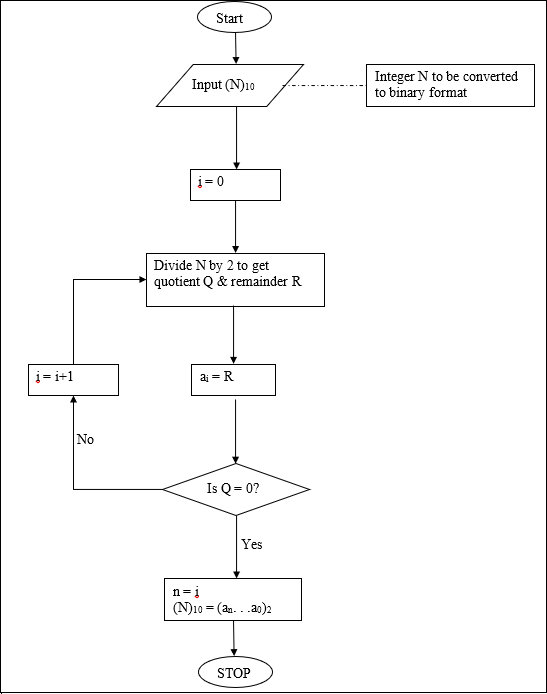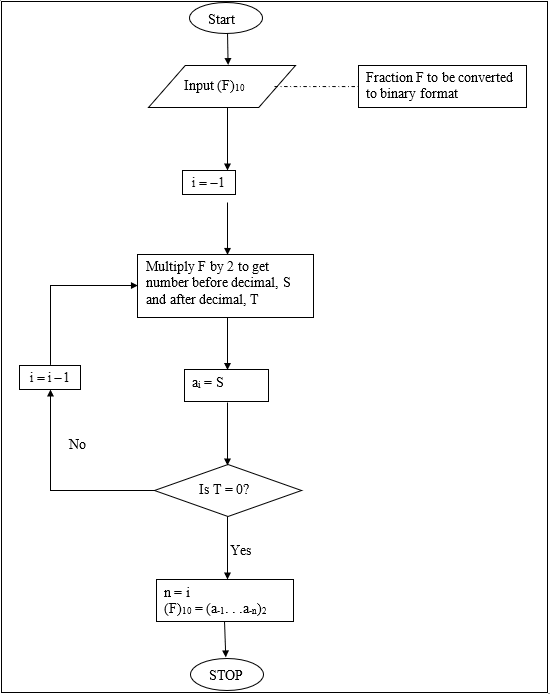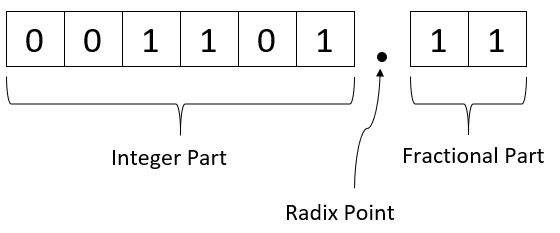# Chapter 01.04: Fixed-Point Binary Representation of Numbers

## Learning Objectives

After successful completion of this lesson, you should be able to:

1) enumerate different bases of number systems and be able to convert base-2 numbers to base-10 numbers.

## Introduction

In everyday life, we use a number system that has a base of $$10$$ (decimal system). When a number is written in this system, we can quickly decipher it as we see such numbers throughout the day.

For example, look at the number $$257.36$$. Each digit in $$257.36$$ has a value of $$0$$ through $$9$$ and has a place value where the position of the number with respect to the decimal point determines its value. Each position in the decimal number corresponds to an integer exponent of $$10$$. In the number $$257.36$$, the $$2$$ is in the place value of hundred, that is $$10^{2}$$, while the $$3$$ is in the place of one-tenths, that is, $$10^{- 1}$$. The number $$257.36$$ can be written as

$\begin{split} 257.36 &= 2\times10^{2} +5\times10^{1}{+7\times}10^{0}{+3\times}10^{- 1}{+6\times}10^{- 2}\\ &= 200 + 50 + 7 + 0.3 + 0.06\\ &= 257.36\end{split}$

In a binary system, we have a similar system as the base-$$10$$, but the only difference is that the base is made of only two digits $$0$$ and $$1$$. So it is a base-$$2$$ system. A number like $$(1011.0011)$$ in base-$$2$$ represents an equivalent decimal number as

$\begin{split} (1011.0011)_{2} &= \begin{pmatrix} \left( 1 \times 2^{3} + 0 \times 2^{2} + 1 \times 2^{1} + 1 \times 2^{0} \right) \\ + (0 \times 2^{- 1} + 0 \times 2^{- 2} + 1 \times 2^{- 3} + 1 \times 2^{- 4}) \\ \end{pmatrix}_{10}\\ &= \begin{pmatrix} \left( 1 \times 8 + 0 \times 4 + 1 \times 2 + 1 \times 1 \right) \\ + (0 \times 0.5 + 0 \times 0.25 + 1 \times 0.125 + 1 \times 0.0625) \\ \end{pmatrix}_{10}\\ &= \left( 8 + 0 + 2 + 1 + 0 + 0 + 0.125 + 0.0625 \right)_{10}\\ &= \left( 11.1875 \right)_{10}\end{split}$

Note the dot (.) that separates the integer and fractional part in the binary representation or any other representation is called the radix point.

### Example 1

Find the equivalent of $$\left( 1101 \right)_{2}$$ in base-$$10$$.

Solution

$\begin{split} (1101)_{2} &= \left( 1 \times 2^{3} + 1 \times 2^{2} + 0 \times 2^{1} + 1 \times 2^{0} \right)_{10}\\ &= \left( 1 \times 8 + 1 \times 4 + 0 \times 2 + 1 \times 1 \right)_{10}\\ &= {\left( 8 + 4 + 0 + 1 \right)}_{10}\\ &= \left( 13 \right)_{10}\end{split}$

### Example 2

Find the equivalent of $$\left( 0.1101 \right)_{2}$$ in base-$$10$$.

Solution

$\begin{split} (0.1101)_{2} &= \left( 1 \times 2^{- 1} + 1 \times 2^{- 2} + 0 \times 2^{- 3} + 1 \times 2^{- 4} \right)_{10}\\ &= \left( 0.5 + 0.25 + 0 + 0.0625 \right)_{10}\\ &= \left( 0.8125 \right)_{10}\end{split}$

### Example 3

Find the equivalent of $$\left( 1101.1101 \right)_{2}$$ in base-$$10$$.

Solution

From Example 1,

$(1101)_{2} = \left( 13 \right)_{10}$

From Example 2,

$(0.1101)_{2} = \left( 0.8125 \right)_{10}$

Hence

$(1101.1101)_{2} = \left( 13.8125 \right)_{10}$

## Learning Objectives

After successful completion of this lesson, you should be able to:

1) convert a base-10 number to a base-2 number in a fixed format.

## Introduction

To understand the binary system, we need to convert binary numbers to decimal numbers and vice-versa. We have already seen an example of how binary numbers can be converted to decimal numbers. Let us see how we can convert a decimal number to a binary number. For example, take the decimal number $$11.1875$$.

First, look at the integer part: $$11$$.

Divide $$11$$ by $$2$$. This division gives a quotient of $$5$$ and a remainder of $$1$$. Since the remainder is $$1$$, $$a_{0} = 1$$.

Divide the quotient $$5$$ by $$2$$. This division gives a quotient of $$2$$ and a remainder of $$1$$. Since the remainder is $$1$$, $$a_{1} = 1$$.

Divide the quotient $$2$$ by $$2$$. This division gives a quotient of $$1$$ and a remainder of $$0$$. Since the remainder is $$0$$, $$a_{2} = 0$$.

Divide the quotient $$1$$ by $$2$$. This division gives a quotient of $$0$$ and a remainder of $$1$$. Since the remainder is $$1$$, $$a_{3} = 1$$.

Since the quotient now is $$0$$, the process is stopped. The above steps are summarized in Table 1.

Table 1 Converting a base-$$10$$ integer to binary representation.

Quotient Remainder
$$11/2$$ $$5$$ $$1 = a_{0}$$
$$5/2$$ $$2$$ $$1 = a_{1}$$
$$2/2$$ $$1$$ $$0 = a_{2}$$
$$1/2$$ $$0$$ $$1 = a_{3}$$

Hence

$\begin{split} (11)_{10} &= (a_{3}a_{2}a_{1}a_{0})_{2}\\ &= (1011)_{2}\end{split}$

For any integer, the algorithm for finding the binary equivalent is given in the flow chart in Figure 1.

Now let us look at the decimal part, that is, $$0.1875$$.

Multiply $$0.1875$$ by $$2$$. This multiplication gives $$0.375$$. The number before the decimal is $$0,$$ and the number after the decimal is $$0.375$$. Since the number before the decimal is $$0$$, $$a_{- 1} = 0$$.

Multiply the number after the decimal, that is, $$0.375$$ by $$2$$. This multiplication gives $$0.75$$. The number before the decimal is $$0,$$ and the number after the decimal is $$0.75$$. Since the number before the decimal is $$0$$, $$a_{- 2} = 0$$.

Multiply the number after the decimal, that is, $$0.75$$ by $$2$$. This multiplication gives $$1.5$$. The number before the decimal is $$1,$$ and the number after the decimal is $$0.5$$. Since the number before the decimal is $$1$$, $$a_{- 3} = 1$$.

Multiply the number after the decimal, that is, $$0.5$$ by $$2$$. This multiplication gives $$1.0$$. The number before the decimal is $$1,$$ and the number after the decimal is $$0$$. Since the number before the decimal is $$1$$, $$a_{- 4} = 1.$$

Since the number after the decimal is $$0$$, the conversion is complete. The above steps are summarized in Table 2.Figure 1. A flow chart to convert an integer from decimal to binary format

Table 2. Converting a base-$$10$$ fraction to binary representation.

Number

Number after

decimal

Number before

decimal
$$0.1875\times2$$ $$0.375$$ $$0.375$$ $$0 = a_{- 1}$$
$$0.375\times2$$ $$0.75$$ $$0.75$$ $$0 = a_{- 2}$$
$$0.75\times2$$ $$1.5$$ $$0.5$$ $$1 = a_{- 3}$$
$$0.5\times2$$ $$1.0$$ $$0.0$$ $$1 = a_{- 4}$$

Hence

$\begin{split} (0.1875)_{10} &= (a_{- 1}a_{- 2}a_{- 3}a_{- 4})_{2}\\ &= (0.0011)_{2}\end{split}$

The algorithm for any fraction is given in a flowchart in Figure 2.

Having calculated

$(11)_{10} = (1011)_{2}$

and

$(0.1875)_{10} = (0.0011)_{2},$

we have

$(11.1875)_{10} = (1011.0011)_{2}.$Figure 2. A flow chart to convert a fractional part from decimal to binary format

In the above example, when we were converting the fractional part of the number, we were left with $$0$$ after the decimal number and used that as a place to stop. In many cases, we are never left with a $$0$$ after the decimal number. For example, finding the binary equivalent of $$0.3$$ is summarized in Table 3.

Table 3. Converting a base-$$10$$ fraction to approximate binary representation.

Number

Number after

decimal

Number before

decimal
$$0.3\times2$$
$$0.6$$
$$0.6$$
$$0 = a_{- 1}$$
$$0.6\times2$$
$$1.2$$
$$0.2$$
$$1 = a_{- 2}$$
$$0.2\times2$$
$$0.4$$
$$0.4$$
$$0 = a_{- 3}$$
$$0.4\times2$$
$$0.8$$
$$0.8$$
$$0 = a_{- 4}$$
$$0.8\times2$$
$$1.6$$
$$0.6$$
$$1 = a_{- 5}$$

As you can see, the process will never end. In this case, the number can only be approximated in binary format, that is,

$\begin{split} (0.3)_{10} &\approx (a_{- 1}a_{- 2}a_{- 3}a_{- 4}a_{- 5})_{2}\\ &= (0.01001)_{2}\end{split}$

### Example 1

Convert $$(13.875)_{10}$$ to base $$2$$.

Solution

For $$(13)_{10}$$, conversion to binary format is shown in Table 4.

Table 4. Conversion of base-$$10$$ integer to binary format.

Quotient Remainder
$$13/2$$ $$6$$ $$1 = a_{0}$$
$$6/2$$ $$3$$ $$0 = a_{1}$$
$$3/2$$ $$1$$ $$1 = a_{2}$$
$$1/2$$ $$0$$ $$1 = a_{3}$$

So

$(13)_{10} = (1101)_{2}.$

Conversion of $$(0.875)_{10}$$ to binary format is shown in Table 5.

Table 5. Converting a base-$$10$$ fraction to binary representation.

Number Number after decimal Number before decimal
$$0.875\times2$$ $$1.75$$ $$0.75$$ $$1 = a_{- 1}$$
$$0.75\times 2$$ $$1.5$$ $$0.5$$ $$1 = a_{- 2}$$
$$0.5\times2$$ $$1.0$$ $$0.0$$ $$1 = a_{- 3}$$

So

$(0.875)_{10} = (0.111)_{2}$

Hence

$(13.875)_{10} = (1101.111)_{2}$

## But what is the mathematics behinds this process of converting a decimal number to binary format?

Let $$z$$ be the decimal number written as

$z = x.y$

where

$$x$$ is the integer part, and $$y$$ is the fractional part.

We want to find the binary equivalent of $$x$$. So, we can write

$x = a_{n}2^{n} + a_{n - 1}2^{n - 1} + ... + a_{0}2^{0}$

If we can now find $$a_{0},...,a_{n}$$in the above equation, then

$(x)_{10} = (a_{n}a_{n - 1}\text{...}a_{0})_{2}$

We now want to find the binary equivalent of $$y$$. So, we can write

$y = b_{- 1}2^{- 1} + b_{- 2}2^{- 2} + ... + b_{- m}2^{- m}$

If we can now find $$b_{- 1},...,b_{- m}$$in the above equation, then

$(y)_{10} = (b_{- 1}b_{- 2}\text{...}b_{- m})_{2}$

Let us look at this using the same example as before.

### Example 2

Convert $$(11.1875)_{10}$$ to base $$2$$.

Solution

To convert $$(11)_{10}$$ to base $$2$$, what is the highest power of $$2$$ that is part of $$11$$. That power is $$3$$, as $$2^{3} = 8$$ to give

$11 = 2^{3} + 3$

What is the highest power of $$2$$ that is part of $$3$$? That power is $$1$$, as $$2^{1} = 2$$ to give

$3 = 2^{1} + 1$

So

$\begin{split} 11 &= 2^{3} + 3\\ &= 2^{3} + 2^{1} + 1\end{split}$

What is the highest power of $$2$$ that is part of $$1$$? That power is $$0$$, as $$2^{0} = 1$$ to give

$1 = 2^{0}$

Hence

$\begin{split} (11)_{10} &= 2^{3} + 2^{1} + 1\\ &= 2^{3} + 2^{1} + 2^{0}\\ &= 1 \times 2^{3} + 0 \times 2^{2} + 1 \times 2^{1} + 1 \times 2^{0}\\ &= (1011)_{2}\end{split}$

To convert $$(0.1875)_{10}$$ to base $$2$$, we proceed as follows. What is the smallest negative power of $$2$$ that is less than or equal to $$0.1875$$? That power is $$- 3$$ as $$2^{- 3} = 0.125$$.

So

$0.1875 = 2^{- 3} + 0.0625$

What is the next smallest negative power of $$2$$ that is less than or equal to $$0.0625$$? That power is $$- 4$$ as $$2^{- 4} = 0.0625$$.

So

$0.1875 = 2^{- 3} + 2^{- 4}$

Hence

$\begin{split} (0.1875)_{10} &= 2^{- 3} + 0.0625 = 2^{- 3} + 2^{- 4}\\ &= 0 \times 2^{- 1} + 0 \times 2^{- 2} + 1 \times 2^{- 3} + 1 \times 2^{- 4}\\ &= (0.0011)_{2}\end{split}$

Since

$(11)_{10} = (1011)_{2}$

and

$(0.1875)_{10} = (0.0011)_{2}$

we get

$(11.1875)_{10} = (1011.0011)_{2}$

One can show the above process algebraically for any general number, but it is out-of-scope for this course.

## Learning Objectives

After successful completion of this lesson, you should be able to:

1) write a base-2 number in a given word length.

## Introduction

A fixed-point binary number may need to be stored in a given number of bits called the word length. Some of the bits in the word would be used for the integer part and the rest of them for the fractional part. In addition, a bit would also be used to signify the sign of the number. This is best illustrated through an example.

### Example 1

Given that a fixed-point positive binary number is stored in eight bits word length, where the first six bits are used for the integer part and the next two for the fractional part. How would the $$(13.875)_{10}$$ be stored? Given that $$(13.875)_{10} = (1101.111)_{2}$$

Solution

Since there are six bits for the integer part and two for the fractional part, we will first rewrite the number as

$\begin{split} (13.875)_{10} &= (1101.111)_{2}\\ &\approx (001101.11)_{2}\end{split}$

This occupies the right number of bits for the integer part ($$6 \ \text{bits}$$) and the fractional part ($$2\ \text{bits}$$). We write $$1101$$ as $$001101$$ to occupy the $$6$$ places without changing it value. We approximate the fractional part $$.111$$ to $$.11$$ as we have only $$2$$ bits for it.Figure 1. A fixed-point format.

## Multiple Choice Test

(1). $$\left( 25 \right)_{10} = \left( ? \right)_{2}$$

(A) $$100110$$

(B) $$10011$$

(C) $$11001$$

(D) $$110010$$

(2). $$\left( 1101 \right)_{2} = \left( ? \right)_{10}$$

(A) $$3$$

(B) $$13$$

(C) $$15$$

(D) $$26$$

(3). $$\left( 25.375 \right)_{10} = \left( ?.? \right)_{2}$$

(A) $$100110.011$$

(B) $$11001.011$$

(C) $$10011.0011$$

(D) $$10011.110$$

(4). Representing $$\sqrt{2}$$ in a fixed-point binary register with $$2$$ bits for the integer part and $$3$$ bits for

the fractional part gives a round-off error of most nearly

(A) $$-0.085709$$

(B) $$0.0392$$

(C) $$0.1642$$

(D) $$0.2892$$

(5). An engineer working for the Department of Defense is writing a program that transfers non-negative real numbers to integer format. To avoid overflow problems, the maximum non-negative integer that can be represented in a $$5$$-bit binary integer word is

(A) $$16$$

(B) $$31$$

(C) $$63$$

(D) $$64$$

(6). For a numerically controlled machine, integers need to be stored in a memory location. The minimum number of bits needed for an integer binary word to represent all integers between $$0$$ and $$1024$$ is

(A) $$8$$

(B) $$9$$

(C) $$10$$

(D) $$11$$

For complete solution, go to

## Problem Set

(1). Convert the following
a)  $$(19)_{10} = (?)_{2}$$
b)  $$(75)_{10} = (?)_{2}$$

Answer: $$a)\ 100111\ \ b)\ 1001011$$

(2). Convert the following
a) $$(110111)_{2} = (?)_{10}$$
b) $$(11001)_{2} = (?)_{10}$$

Answer: $$a)\ 55\ \ b)\ 25$$

(3). Convert the following
a) $$(0.375)_{10} = (?)_{2}$$
b) $$(0.075)_{10} = (?)_{2}$$

Answer: $$a)\ 0.011\ \ b)\ 0.000100110011...$$

(4). Convert the following
a) $$(0.110001)_{2} = (0.?)_{10}$$
b)  $$(0.0111)_{2} = (0.?)_{10}$$

Answer: $$a)\ 0.765625\ \ b)\ 0.4375$$

(5). Convert the following
a)  $$(19.375)_{10} = (?.?)_{2}$$
b)  $$(75.075)_{10} = (?.?)_{2}$$

Answer: $$a)\ 10011.011\ \ b)\ 1001011.00010011.......$$

(6). Convert the following
a)  $$(110111.110001)_{2} = (?.?)_{10}$$
b)  $$(11001.0111)_{2} = (?.?)_{10}$$

Answer: $$a)\ 55.765625\ \ b)\ 25.4375$$